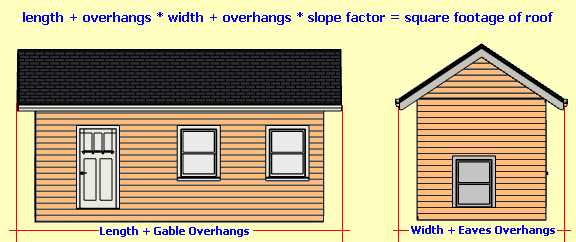Spike's Calculators

# Measuring a Roof

Calculate the square footage of a roof, or how many squares is your roof? A roofing square measures 10 by 10 feet, covering an area of 100 square feet.

A simple process which will not require having to take measurements on top of the roof. This method works well for gable and hip roofs and calculates the square footage of a roof. Once you know the square footage it is easy to calculate the amount of plywood, felt, shingles, or any other roofing materials needed.##### Example calculation:
slope = 6 : 12 = 1.1180 slope factor
building length = 40'
building width = 36'
eave overhang = 24"
gable overhang = 12"

building length + gable overhangs = 42'
building width + eaves = 40'

42 * 40 * 1.1180 = 1,878.24 sq ft

This roof has an area of 1878.24 ft²

Divide this by 100 to get the amount of roofing squares.
1878.24 ÷ 100 = 18.78
Number of squares = 18.8

### Roof Calculator

Building Length ft in
Building Width ft in
Eave Overhang inch
Gable Overhang inch
Roof Pitch /12

#### Results:

 Roof Area ft² Roof Area in Number of Squares sq

#### Calculation

1. building length in feet and inches
2. building width in feet and inches
3. overhang of eave in inches
4. gable overhang in inches
5. roof pitch inches per foot

#### Results

1. square footage
2. number of squares